# General Information¶

The `minerl` package includes several environments as follows. This page describes each of the included environments, provides usage samples, and describes the exact action and observation space provided by each environment!

Caution

In the MineRL Competition, many environments are provided for training, however competition agents will only be evaluated in `MineRLObtainDiamondVectorObf-v0` which has sparse rewards. See MineRLObtainDiamondVectorObf-v0.

Note

All environments offer a default no-op action via `env.action_space.no_op()` and a random action via `env.action_space.sample()`.

# Environment Handlers¶

Minecraft is an extremely complex environment which provides players with visual, auditory, and informational observation of many complex data types. Furthermore, players interact with Minecraft using more than just embodied actions: players can craft, build, destroy, smelt, enchant, manage their inventory, and even communicate with other players via a text chat.

To provide a unified interface with which agents can obtain and perform similar observations and actions as players, we have provided first-class for support for this multi-modality in the environment: the observation and action spaces of environments are `gym.spaces.Dict` spaces. These observation and action dictionaries are comprised of individual fields we call handlers.

Note

In the documentation of every environment we provide a listing of the exact `gym.space` of the observations returned by and actions expected by the environment’s step function. We are slowly building documentation for these handlers, and you can click those highlighted with blue for more information!

# Basic Environments¶

Warning

The following Basic Environments are NOT part of the MineRL Diamond and BASALT competitions!

Feel free to use them for personal exploration, but note that competitions agents may only be trained on their corresponding competition environments.

## MineRLTreechop-v0¶In treechop, the agent must collect 64 minecraft:log. This replicates a common scenario in Minecraft, as logs are necessary to craft a large amount of items in the game and are a key resource in Minecraft.

The agent begins in a forest biome (near many trees) with an iron axe for cutting trees. The agent is given +1 reward for obtaining each unit of wood, and the episode terminates once the agent obtains 64 units.

### Observation Space¶

```Dict({
"pov": "Box(low=0, high=255, shape=(64, 64, 3))"
})```

### Action Space¶

```Dict({
"attack": "Discrete(2)",
"back": "Discrete(2)",
"camera": "Box(low=-180.0, high=180.0, shape=(2,))",
"forward": "Discrete(2)",
"jump": "Discrete(2)",
"left": "Discrete(2)",
"right": "Discrete(2)",
"sneak": "Discrete(2)",
"sprint": "Discrete(2)"
})```

### Usage¶

```import gym
import minerl

# Run a random agent through the environment
env = gym.make("MineRLTreechop-v0")  # A MineRLTreechop-v0 env

obs = env.reset()
done = False

while not done:
# Take a no-op through the environment.
obs, rew, done, _ = env.step(env.action_space.noop())
# Do something

######################################

# Sample some data from the dataset!
data = minerl.data.make("MineRLTreechop-v0")

# Iterate through a single epoch using sequences of at most 32 steps
for obs, rew, done, act in data.batch_iter(num_epochs=1, batch_size=32):
# Do something
```

## MineRLNavigate-v0¶In this task, the agent must move to a goal location denoted by a diamond block. This represents a basic primitive used in many tasks throughout Minecraft. In addition to standard observations, the agent has access to a “compass” observation, which points near the goal location, 64 meters from the start location. The goal has a small random horizontal offset from the compass location and may be slightly below surface level. On the goal location is a unique block, so the agent must find the final goal by searching based on local visual features.

The agent is given a sparse reward (+100 upon reaching the goal, at which point the episode terminates). This variant of the environment is sparse.

In this environment, the agent spawns on a random survival map.

### Observation Space¶

```Dict({
"compass": {
"angle": "Box(low=-180.0, high=180.0, shape=())"
},
"inventory": {
"dirt": "Box(low=0, high=2304, shape=())"
},
"pov": "Box(low=0, high=255, shape=(64, 64, 3))"
})```

### Action Space¶

```Dict({
"attack": "Discrete(2)",
"back": "Discrete(2)",
"camera": "Box(low=-180.0, high=180.0, shape=(2,))",
"forward": "Discrete(2)",
"jump": "Discrete(2)",
"left": "Discrete(2)",
"place": "Enum(dirt,none)",
"right": "Discrete(2)",
"sneak": "Discrete(2)",
"sprint": "Discrete(2)"
})```

### Usage¶

```import gym
import minerl

# Run a random agent through the environment
env = gym.make("MineRLNavigate-v0")  # A MineRLNavigate-v0 env

obs = env.reset()
done = False

while not done:
# Take a no-op through the environment.
obs, rew, done, _ = env.step(env.action_space.noop())
# Do something

######################################

# Sample some data from the dataset!
data = minerl.data.make("MineRLNavigate-v0")

# Iterate through a single epoch using sequences of at most 32 steps
for obs, rew, done, act in data.batch_iter(num_epochs=1, batch_size=32):
# Do something
```

## MineRLNavigateDense-v0¶In this task, the agent must move to a goal location denoted by a diamond block. This represents a basic primitive used in many tasks throughout Minecraft. In addition to standard observations, the agent has access to a “compass” observation, which points near the goal location, 64 meters from the start location. The goal has a small random horizontal offset from the compass location and may be slightly below surface level. On the goal location is a unique block, so the agent must find the final goal by searching based on local visual features.

The agent is given a sparse reward (+100 upon reaching the goal, at which point the episode terminates). This variant of the environment is dense reward-shaped where the agent is given a reward every tick for how much closer (or negative reward for farther) the agent gets to the target.

In this environment, the agent spawns on a random survival map.

### Observation Space¶

```Dict({
"compass": {
"angle": "Box(low=-180.0, high=180.0, shape=())"
},
"inventory": {
"dirt": "Box(low=0, high=2304, shape=())"
},
"pov": "Box(low=0, high=255, shape=(64, 64, 3))"
})```

### Action Space¶

```Dict({
"attack": "Discrete(2)",
"back": "Discrete(2)",
"camera": "Box(low=-180.0, high=180.0, shape=(2,))",
"forward": "Discrete(2)",
"jump": "Discrete(2)",
"left": "Discrete(2)",
"place": "Enum(dirt,none)",
"right": "Discrete(2)",
"sneak": "Discrete(2)",
"sprint": "Discrete(2)"
})```

### Usage¶

```import gym
import minerl

# Run a random agent through the environment
env = gym.make("MineRLNavigateDense-v0")  # A MineRLNavigateDense-v0 env

obs = env.reset()
done = False

while not done:
# Take a no-op through the environment.
obs, rew, done, _ = env.step(env.action_space.noop())
# Do something

######################################

# Sample some data from the dataset!
data = minerl.data.make("MineRLNavigateDense-v0")

# Iterate through a single epoch using sequences of at most 32 steps
for obs, rew, done, act in data.batch_iter(num_epochs=1, batch_size=32):
# Do something
```

## MineRLNavigateExtreme-v0¶In this task, the agent must move to a goal location denoted by a diamond block. This represents a basic primitive used in many tasks throughout Minecraft. In addition to standard observations, the agent has access to a “compass” observation, which points near the goal location, 64 meters from the start location. The goal has a small random horizontal offset from the compass location and may be slightly below surface level. On the goal location is a unique block, so the agent must find the final goal by searching based on local visual features.

The agent is given a sparse reward (+100 upon reaching the goal, at which point the episode terminates). This variant of the environment is sparse.

In this environment, the agent spawns in an extreme hills biome.

### Observation Space¶

```Dict({
"compass": {
"angle": "Box(low=-180.0, high=180.0, shape=())"
},
"inventory": {
"dirt": "Box(low=0, high=2304, shape=())"
},
"pov": "Box(low=0, high=255, shape=(64, 64, 3))"
})```

### Action Space¶

```Dict({
"attack": "Discrete(2)",
"back": "Discrete(2)",
"camera": "Box(low=-180.0, high=180.0, shape=(2,))",
"forward": "Discrete(2)",
"jump": "Discrete(2)",
"left": "Discrete(2)",
"place": "Enum(dirt,none)",
"right": "Discrete(2)",
"sneak": "Discrete(2)",
"sprint": "Discrete(2)"
})```

### Usage¶

```import gym
import minerl

# Run a random agent through the environment
env = gym.make("MineRLNavigateExtreme-v0")  # A MineRLNavigateExtreme-v0 env

obs = env.reset()
done = False

while not done:
# Take a no-op through the environment.
obs, rew, done, _ = env.step(env.action_space.noop())
# Do something

######################################

# Sample some data from the dataset!
data = minerl.data.make("MineRLNavigateExtreme-v0")

# Iterate through a single epoch using sequences of at most 32 steps
for obs, rew, done, act in data.batch_iter(num_epochs=1, batch_size=32):
# Do something
```

## MineRLNavigateExtremeDense-v0¶In this task, the agent must move to a goal location denoted by a diamond block. This represents a basic primitive used in many tasks throughout Minecraft. In addition to standard observations, the agent has access to a “compass” observation, which points near the goal location, 64 meters from the start location. The goal has a small random horizontal offset from the compass location and may be slightly below surface level. On the goal location is a unique block, so the agent must find the final goal by searching based on local visual features.

The agent is given a sparse reward (+100 upon reaching the goal, at which point the episode terminates). This variant of the environment is dense reward-shaped where the agent is given a reward every tick for how much closer (or negative reward for farther) the agent gets to the target.

In this environment, the agent spawns in an extreme hills biome.

### Observation Space¶

```Dict({
"compass": {
"angle": "Box(low=-180.0, high=180.0, shape=())"
},
"inventory": {
"dirt": "Box(low=0, high=2304, shape=())"
},
"pov": "Box(low=0, high=255, shape=(64, 64, 3))"
})```

### Action Space¶

```Dict({
"attack": "Discrete(2)",
"back": "Discrete(2)",
"camera": "Box(low=-180.0, high=180.0, shape=(2,))",
"forward": "Discrete(2)",
"jump": "Discrete(2)",
"left": "Discrete(2)",
"place": "Enum(dirt,none)",
"right": "Discrete(2)",
"sneak": "Discrete(2)",
"sprint": "Discrete(2)"
})```

### Usage¶

```import gym
import minerl

# Run a random agent through the environment
env = gym.make("MineRLNavigateExtremeDense-v0")  # A MineRLNavigateExtremeDense-v0 env

obs = env.reset()
done = False

while not done:
# Take a no-op through the environment.
obs, rew, done, _ = env.step(env.action_space.noop())
# Do something

######################################

# Sample some data from the dataset!
data = minerl.data.make("MineRLNavigateExtremeDense-v0")

# Iterate through a single epoch using sequences of at most 32 steps
for obs, rew, done, act in data.batch_iter(num_epochs=1, batch_size=32):
# Do something
```

## MineRLObtainDiamond-v0¶In this environment the agent is required to obtain a diamond. The agent begins in a random starting location on a random survival map without any items, matching the normal starting conditions for human players in Minecraft. The agent is given access to a selected summary of its inventory and GUI free crafting, smelting, and inventory management actions.

During an episode the agent is rewarded every time it obtains an item in the requisite item hierarchy to obtaining a diamond. The rewards for each item are given here:

```<Item reward="1" type="log" />
<Item reward="2" type="planks" />
<Item reward="4" type="stick" />
<Item reward="4" type="crafting_table" />
<Item reward="8" type="wooden_pickaxe" />
<Item reward="16" type="cobblestone" />
<Item reward="32" type="furnace" />
<Item reward="32" type="stone_pickaxe" />
<Item reward="64" type="iron_ore" />
<Item reward="128" type="iron_ingot" />
<Item reward="256" type="iron_pickaxe" />
<Item reward="1024" type="diamond" />
```

### Observation Space¶

```Dict({
"equipped_items": {
"mainhand": {
"damage": "Box(low=-1, high=1562, shape=())",
"maxDamage": "Box(low=-1, high=1562, shape=())",
"type": "Enum(air,iron_axe,iron_pickaxe,none,other,stone_axe,stone_pickaxe,wooden_axe,wooden_pickaxe)"
}
},
"inventory": {
"coal": "Box(low=0, high=2304, shape=())",
"cobblestone": "Box(low=0, high=2304, shape=())",
"crafting_table": "Box(low=0, high=2304, shape=())",
"dirt": "Box(low=0, high=2304, shape=())",
"furnace": "Box(low=0, high=2304, shape=())",
"iron_axe": "Box(low=0, high=2304, shape=())",
"iron_ingot": "Box(low=0, high=2304, shape=())",
"iron_ore": "Box(low=0, high=2304, shape=())",
"iron_pickaxe": "Box(low=0, high=2304, shape=())",
"log": "Box(low=0, high=2304, shape=())",
"planks": "Box(low=0, high=2304, shape=())",
"stick": "Box(low=0, high=2304, shape=())",
"stone": "Box(low=0, high=2304, shape=())",
"stone_axe": "Box(low=0, high=2304, shape=())",
"stone_pickaxe": "Box(low=0, high=2304, shape=())",
"torch": "Box(low=0, high=2304, shape=())",
"wooden_axe": "Box(low=0, high=2304, shape=())",
"wooden_pickaxe": "Box(low=0, high=2304, shape=())"
},
"pov": "Box(low=0, high=255, shape=(64, 64, 3))"
})```

### Action Space¶

```Dict({
"attack": "Discrete(2)",
"back": "Discrete(2)",
"camera": "Box(low=-180.0, high=180.0, shape=(2,))",
"craft": "Enum(crafting_table,none,planks,stick,torch)",
"equip": "Enum(air,iron_axe,iron_pickaxe,none,stone_axe,stone_pickaxe,wooden_axe,wooden_pickaxe)",
"forward": "Discrete(2)",
"jump": "Discrete(2)",
"left": "Discrete(2)",
"nearbyCraft": "Enum(furnace,iron_axe,iron_pickaxe,none,stone_axe,stone_pickaxe,wooden_axe,wooden_pickaxe)",
"nearbySmelt": "Enum(coal,iron_ingot,none)",
"place": "Enum(cobblestone,crafting_table,dirt,furnace,none,stone,torch)",
"right": "Discrete(2)",
"sneak": "Discrete(2)",
"sprint": "Discrete(2)"
})```

### Usage¶

```import gym
import minerl

# Run a random agent through the environment
env = gym.make("MineRLObtainDiamond-v0")  # A MineRLObtainDiamond-v0 env

obs = env.reset()
done = False

while not done:
# Take a no-op through the environment.
obs, rew, done, _ = env.step(env.action_space.noop())
# Do something

######################################

# Sample some data from the dataset!
data = minerl.data.make("MineRLObtainDiamond-v0")

# Iterate through a single epoch using sequences of at most 32 steps
for obs, rew, done, act in data.batch_iter(num_epochs=1, batch_size=32):
# Do something
```

## MineRLObtainDiamondDense-v0¶In this environment the agent is required to obtain a diamond. The agent begins in a random starting location on a random survival map without any items, matching the normal starting conditions for human players in Minecraft. The agent is given access to a selected summary of its inventory and GUI free crafting, smelting, and inventory management actions.

During an episode the agent is rewarded every time it obtains an item in the requisite item hierarchy to obtaining a diamond. The rewards for each item are given here:

```<Item reward="1" type="log" />
<Item reward="2" type="planks" />
<Item reward="4" type="stick" />
<Item reward="4" type="crafting_table" />
<Item reward="8" type="wooden_pickaxe" />
<Item reward="16" type="cobblestone" />
<Item reward="32" type="furnace" />
<Item reward="32" type="stone_pickaxe" />
<Item reward="64" type="iron_ore" />
<Item reward="128" type="iron_ingot" />
<Item reward="256" type="iron_pickaxe" />
<Item reward="1024" type="diamond" />
```

### Observation Space¶

```Dict({
"equipped_items": {
"mainhand": {
"damage": "Box(low=-1, high=1562, shape=())",
"maxDamage": "Box(low=-1, high=1562, shape=())",
"type": "Enum(air,iron_axe,iron_pickaxe,none,other,stone_axe,stone_pickaxe,wooden_axe,wooden_pickaxe)"
}
},
"inventory": {
"coal": "Box(low=0, high=2304, shape=())",
"cobblestone": "Box(low=0, high=2304, shape=())",
"crafting_table": "Box(low=0, high=2304, shape=())",
"dirt": "Box(low=0, high=2304, shape=())",
"furnace": "Box(low=0, high=2304, shape=())",
"iron_axe": "Box(low=0, high=2304, shape=())",
"iron_ingot": "Box(low=0, high=2304, shape=())",
"iron_ore": "Box(low=0, high=2304, shape=())",
"iron_pickaxe": "Box(low=0, high=2304, shape=())",
"log": "Box(low=0, high=2304, shape=())",
"planks": "Box(low=0, high=2304, shape=())",
"stick": "Box(low=0, high=2304, shape=())",
"stone": "Box(low=0, high=2304, shape=())",
"stone_axe": "Box(low=0, high=2304, shape=())",
"stone_pickaxe": "Box(low=0, high=2304, shape=())",
"torch": "Box(low=0, high=2304, shape=())",
"wooden_axe": "Box(low=0, high=2304, shape=())",
"wooden_pickaxe": "Box(low=0, high=2304, shape=())"
},
"pov": "Box(low=0, high=255, shape=(64, 64, 3))"
})```

### Action Space¶

```Dict({
"attack": "Discrete(2)",
"back": "Discrete(2)",
"camera": "Box(low=-180.0, high=180.0, shape=(2,))",
"craft": "Enum(crafting_table,none,planks,stick,torch)",
"equip": "Enum(air,iron_axe,iron_pickaxe,none,stone_axe,stone_pickaxe,wooden_axe,wooden_pickaxe)",
"forward": "Discrete(2)",
"jump": "Discrete(2)",
"left": "Discrete(2)",
"nearbyCraft": "Enum(furnace,iron_axe,iron_pickaxe,none,stone_axe,stone_pickaxe,wooden_axe,wooden_pickaxe)",
"nearbySmelt": "Enum(coal,iron_ingot,none)",
"place": "Enum(cobblestone,crafting_table,dirt,furnace,none,stone,torch)",
"right": "Discrete(2)",
"sneak": "Discrete(2)",
"sprint": "Discrete(2)"
})```

### Usage¶

```import gym
import minerl

# Run a random agent through the environment
env = gym.make("MineRLObtainDiamondDense-v0")  # A MineRLObtainDiamondDense-v0 env

obs = env.reset()
done = False

while not done:
# Take a no-op through the environment.
obs, rew, done, _ = env.step(env.action_space.noop())
# Do something

######################################

# Sample some data from the dataset!
data = minerl.data.make("MineRLObtainDiamondDense-v0")

# Iterate through a single epoch using sequences of at most 32 steps
for obs, rew, done, act in data.batch_iter(num_epochs=1, batch_size=32):
# Do something
```

## MineRLObtainIronPickaxe-v0¶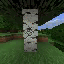In this environment the agent is required to obtain an iron pickaxe. The agent begins in a random starting location, on a random survival map, without any items, matching the normal starting conditions for human players in Minecraft. The agent is given access to a selected view of its inventory and GUI free crafting, smelting, and inventory management actions.

During an episode the agent is rewarded only once per item the first time it obtains that item in the requisite item hierarchy for obtaining an iron pickaxe. The reward for each item is given here:

```<Item amount="1" reward="1" type="log" />
<Item amount="1" reward="2" type="planks" />
<Item amount="1" reward="4" type="stick" />
<Item amount="1" reward="4" type="crafting_table" />
<Item amount="1" reward="8" type="wooden_pickaxe" />
<Item amount="1" reward="16" type="cobblestone" />
<Item amount="1" reward="32" type="furnace" />
<Item amount="1" reward="32" type="stone_pickaxe" />
<Item amount="1" reward="64" type="iron_ore" />
<Item amount="1" reward="128" type="iron_ingot" />
<Item amount="1" reward="256" type="iron_pickaxe" />
```

### Observation Space¶

```Dict({
"equipped_items": {
"mainhand": {
"damage": "Box(low=-1, high=1562, shape=())",
"maxDamage": "Box(low=-1, high=1562, shape=())",
"type": "Enum(air,iron_axe,iron_pickaxe,none,other,stone_axe,stone_pickaxe,wooden_axe,wooden_pickaxe)"
}
},
"inventory": {
"coal": "Box(low=0, high=2304, shape=())",
"cobblestone": "Box(low=0, high=2304, shape=())",
"crafting_table": "Box(low=0, high=2304, shape=())",
"dirt": "Box(low=0, high=2304, shape=())",
"furnace": "Box(low=0, high=2304, shape=())",
"iron_axe": "Box(low=0, high=2304, shape=())",
"iron_ingot": "Box(low=0, high=2304, shape=())",
"iron_ore": "Box(low=0, high=2304, shape=())",
"iron_pickaxe": "Box(low=0, high=2304, shape=())",
"log": "Box(low=0, high=2304, shape=())",
"planks": "Box(low=0, high=2304, shape=())",
"stick": "Box(low=0, high=2304, shape=())",
"stone": "Box(low=0, high=2304, shape=())",
"stone_axe": "Box(low=0, high=2304, shape=())",
"stone_pickaxe": "Box(low=0, high=2304, shape=())",
"torch": "Box(low=0, high=2304, shape=())",
"wooden_axe": "Box(low=0, high=2304, shape=())",
"wooden_pickaxe": "Box(low=0, high=2304, shape=())"
},
"pov": "Box(low=0, high=255, shape=(64, 64, 3))"
})```

### Action Space¶

```Dict({
"attack": "Discrete(2)",
"back": "Discrete(2)",
"camera": "Box(low=-180.0, high=180.0, shape=(2,))",
"craft": "Enum(crafting_table,none,planks,stick,torch)",
"equip": "Enum(air,iron_axe,iron_pickaxe,none,stone_axe,stone_pickaxe,wooden_axe,wooden_pickaxe)",
"forward": "Discrete(2)",
"jump": "Discrete(2)",
"left": "Discrete(2)",
"nearbyCraft": "Enum(furnace,iron_axe,iron_pickaxe,none,stone_axe,stone_pickaxe,wooden_axe,wooden_pickaxe)",
"nearbySmelt": "Enum(coal,iron_ingot,none)",
"place": "Enum(cobblestone,crafting_table,dirt,furnace,none,stone,torch)",
"right": "Discrete(2)",
"sneak": "Discrete(2)",
"sprint": "Discrete(2)"
})```

### Usage¶

```import gym
import minerl

# Run a random agent through the environment
env = gym.make("MineRLObtainIronPickaxe-v0")  # A MineRLObtainIronPickaxe-v0 env

obs = env.reset()
done = False

while not done:
# Take a no-op through the environment.
obs, rew, done, _ = env.step(env.action_space.noop())
# Do something

######################################

# Sample some data from the dataset!
data = minerl.data.make("MineRLObtainIronPickaxe-v0")

# Iterate through a single epoch using sequences of at most 32 steps
for obs, rew, done, act in data.batch_iter(num_epochs=1, batch_size=32):
# Do something
```

## MineRLObtainIronPickaxeDense-v0¶In this environment the agent is required to obtain an iron pickaxe. The agent begins in a random starting location, on a random survival map, without any items, matching the normal starting conditions for human players in Minecraft. The agent is given access to a selected view of its inventory and GUI free crafting, smelting, and inventory management actions.

During an episode the agent is rewarded only once per item the first time it obtains that item in the requisite item hierarchy for obtaining an iron pickaxe. The reward for each item is given here:

```<Item amount="1" reward="1" type="log" />
<Item amount="1" reward="2" type="planks" />
<Item amount="1" reward="4" type="stick" />
<Item amount="1" reward="4" type="crafting_table" />
<Item amount="1" reward="8" type="wooden_pickaxe" />
<Item amount="1" reward="16" type="cobblestone" />
<Item amount="1" reward="32" type="furnace" />
<Item amount="1" reward="32" type="stone_pickaxe" />
<Item amount="1" reward="64" type="iron_ore" />
<Item amount="1" reward="128" type="iron_ingot" />
<Item amount="1" reward="256" type="iron_pickaxe" />
```

### Observation Space¶

```Dict({
"equipped_items": {
"mainhand": {
"damage": "Box(low=-1, high=1562, shape=())",
"maxDamage": "Box(low=-1, high=1562, shape=())",
"type": "Enum(air,iron_axe,iron_pickaxe,none,other,stone_axe,stone_pickaxe,wooden_axe,wooden_pickaxe)"
}
},
"inventory": {
"coal": "Box(low=0, high=2304, shape=())",
"cobblestone": "Box(low=0, high=2304, shape=())",
"crafting_table": "Box(low=0, high=2304, shape=())",
"dirt": "Box(low=0, high=2304, shape=())",
"furnace": "Box(low=0, high=2304, shape=())",
"iron_axe": "Box(low=0, high=2304, shape=())",
"iron_ingot": "Box(low=0, high=2304, shape=())",
"iron_ore": "Box(low=0, high=2304, shape=())",
"iron_pickaxe": "Box(low=0, high=2304, shape=())",
"log": "Box(low=0, high=2304, shape=())",
"planks": "Box(low=0, high=2304, shape=())",
"stick": "Box(low=0, high=2304, shape=())",
"stone": "Box(low=0, high=2304, shape=())",
"stone_axe": "Box(low=0, high=2304, shape=())",
"stone_pickaxe": "Box(low=0, high=2304, shape=())",
"torch": "Box(low=0, high=2304, shape=())",
"wooden_axe": "Box(low=0, high=2304, shape=())",
"wooden_pickaxe": "Box(low=0, high=2304, shape=())"
},
"pov": "Box(low=0, high=255, shape=(64, 64, 3))"
})```

### Action Space¶

```Dict({
"attack": "Discrete(2)",
"back": "Discrete(2)",
"camera": "Box(low=-180.0, high=180.0, shape=(2,))",
"craft": "Enum(crafting_table,none,planks,stick,torch)",
"equip": "Enum(air,iron_axe,iron_pickaxe,none,stone_axe,stone_pickaxe,wooden_axe,wooden_pickaxe)",
"forward": "Discrete(2)",
"jump": "Discrete(2)",
"left": "Discrete(2)",
"nearbyCraft": "Enum(furnace,iron_axe,iron_pickaxe,none,stone_axe,stone_pickaxe,wooden_axe,wooden_pickaxe)",
"nearbySmelt": "Enum(coal,iron_ingot,none)",
"place": "Enum(cobblestone,crafting_table,dirt,furnace,none,stone,torch)",
"right": "Discrete(2)",
"sneak": "Discrete(2)",
"sprint": "Discrete(2)"
})```

### Usage¶

```import gym
import minerl

# Run a random agent through the environment
env = gym.make("MineRLObtainIronPickaxeDense-v0")  # A MineRLObtainIronPickaxeDense-v0 env

obs = env.reset()
done = False

while not done:
# Take a no-op through the environment.
obs, rew, done, _ = env.step(env.action_space.noop())
# Do something

######################################

# Sample some data from the dataset!
data = minerl.data.make("MineRLObtainIronPickaxeDense-v0")

# Iterate through a single epoch using sequences of at most 32 steps
for obs, rew, done, act in data.batch_iter(num_epochs=1, batch_size=32):
# Do something
```

# MineRL Diamond Competition Environments¶

## MineRLTreechopVectorObf-v0¶In treechop, the agent must collect 64 minecraft:log. This replicates a common scenario in Minecraft, as logs are necessary to craft a large amount of items in the game and are a key resource in Minecraft.

The agent begins in a forest biome (near many trees) with an iron axe for cutting trees. The agent is given +1 reward for obtaining each unit of wood, and the episode terminates once the agent obtains 64 units.

### Observation Space¶

```Dict({
"pov": "Box(low=0, high=255, shape=(64, 64, 3))",
"vector": "Box(low=-1.2000000476837158, high=1.2000000476837158, shape=(64,))"
})```

### Action Space¶

```Dict({
"vector": "Box(low=-1.0499999523162842, high=1.0499999523162842, shape=(64,))"
})```

### Usage¶

```import gym
import minerl

# Run a random agent through the environment
env = gym.make("MineRLTreechopVectorObf-v0")  # A MineRLTreechopVectorObf-v0 env

obs = env.reset()
done = False

while not done:
# Take a no-op through the environment.
obs, rew, done, _ = env.step(env.action_space.noop())
# Do something

######################################

# Sample some data from the dataset!
data = minerl.data.make("MineRLTreechopVectorObf-v0")

# Iterate through a single epoch using sequences of at most 32 steps
for obs, rew, done, act in data.batch_iter(num_epochs=1, batch_size=32):
# Do something
```

## MineRLNavigateVectorObf-v0¶In this task, the agent must move to a goal location denoted by a diamond block. This represents a basic primitive used in many tasks throughout Minecraft. In addition to standard observations, the agent has access to a “compass” observation, which points near the goal location, 64 meters from the start location. The goal has a small random horizontal offset from the compass location and may be slightly below surface level. On the goal location is a unique block, so the agent must find the final goal by searching based on local visual features.

The agent is given a sparse reward (+100 upon reaching the goal, at which point the episode terminates). This variant of the environment is sparse.

In this environment, the agent spawns on a random survival map.

### Observation Space¶

```Dict({
"pov": "Box(low=0, high=255, shape=(64, 64, 3))",
"vector": "Box(low=-1.2000000476837158, high=1.2000000476837158, shape=(64,))"
})```

### Action Space¶

```Dict({
"vector": "Box(low=-1.0499999523162842, high=1.0499999523162842, shape=(64,))"
})```

### Usage¶

```import gym
import minerl

# Run a random agent through the environment
env = gym.make("MineRLNavigateVectorObf-v0")  # A MineRLNavigateVectorObf-v0 env

obs = env.reset()
done = False

while not done:
# Take a no-op through the environment.
obs, rew, done, _ = env.step(env.action_space.noop())
# Do something

######################################

# Sample some data from the dataset!
data = minerl.data.make("MineRLNavigateVectorObf-v0")

# Iterate through a single epoch using sequences of at most 32 steps
for obs, rew, done, act in data.batch_iter(num_epochs=1, batch_size=32):
# Do something
```

## MineRLNavigateDenseVectorObf-v0¶In this task, the agent must move to a goal location denoted by a diamond block. This represents a basic primitive used in many tasks throughout Minecraft. In addition to standard observations, the agent has access to a “compass” observation, which points near the goal location, 64 meters from the start location. The goal has a small random horizontal offset from the compass location and may be slightly below surface level. On the goal location is a unique block, so the agent must find the final goal by searching based on local visual features.

The agent is given a sparse reward (+100 upon reaching the goal, at which point the episode terminates). This variant of the environment is dense reward-shaped where the agent is given a reward every tick for how much closer (or negative reward for farther) the agent gets to the target.

In this environment, the agent spawns on a random survival map.

### Observation Space¶

```Dict({
"pov": "Box(low=0, high=255, shape=(64, 64, 3))",
"vector": "Box(low=-1.2000000476837158, high=1.2000000476837158, shape=(64,))"
})```

### Action Space¶

```Dict({
"vector": "Box(low=-1.0499999523162842, high=1.0499999523162842, shape=(64,))"
})```

### Usage¶

```import gym
import minerl

# Run a random agent through the environment
env = gym.make("MineRLNavigateDenseVectorObf-v0")  # A MineRLNavigateDenseVectorObf-v0 env

obs = env.reset()
done = False

while not done:
# Take a no-op through the environment.
obs, rew, done, _ = env.step(env.action_space.noop())
# Do something

######################################

# Sample some data from the dataset!
data = minerl.data.make("MineRLNavigateDenseVectorObf-v0")

# Iterate through a single epoch using sequences of at most 32 steps
for obs, rew, done, act in data.batch_iter(num_epochs=1, batch_size=32):
# Do something
```

## MineRLNavigateExtremeVectorObf-v0¶In this task, the agent must move to a goal location denoted by a diamond block. This represents a basic primitive used in many tasks throughout Minecraft. In addition to standard observations, the agent has access to a “compass” observation, which points near the goal location, 64 meters from the start location. The goal has a small random horizontal offset from the compass location and may be slightly below surface level. On the goal location is a unique block, so the agent must find the final goal by searching based on local visual features.

The agent is given a sparse reward (+100 upon reaching the goal, at which point the episode terminates). This variant of the environment is sparse.

In this environment, the agent spawns in an extreme hills biome.

### Observation Space¶

```Dict({
"pov": "Box(low=0, high=255, shape=(64, 64, 3))",
"vector": "Box(low=-1.2000000476837158, high=1.2000000476837158, shape=(64,))"
})```

### Action Space¶

```Dict({
"vector": "Box(low=-1.0499999523162842, high=1.0499999523162842, shape=(64,))"
})```

### Usage¶

```import gym
import minerl

# Run a random agent through the environment
env = gym.make("MineRLNavigateExtremeVectorObf-v0")  # A MineRLNavigateExtremeVectorObf-v0 env

obs = env.reset()
done = False

while not done:
# Take a no-op through the environment.
obs, rew, done, _ = env.step(env.action_space.noop())
# Do something

######################################

# Sample some data from the dataset!
data = minerl.data.make("MineRLNavigateExtremeVectorObf-v0")

# Iterate through a single epoch using sequences of at most 32 steps
for obs, rew, done, act in data.batch_iter(num_epochs=1, batch_size=32):
# Do something
```

## MineRLNavigateExtremeDenseVectorObf-v0¶In this task, the agent must move to a goal location denoted by a diamond block. This represents a basic primitive used in many tasks throughout Minecraft. In addition to standard observations, the agent has access to a “compass” observation, which points near the goal location, 64 meters from the start location. The goal has a small random horizontal offset from the compass location and may be slightly below surface level. On the goal location is a unique block, so the agent must find the final goal by searching based on local visual features.

The agent is given a sparse reward (+100 upon reaching the goal, at which point the episode terminates). This variant of the environment is dense reward-shaped where the agent is given a reward every tick for how much closer (or negative reward for farther) the agent gets to the target.

In this environment, the agent spawns in an extreme hills biome.

### Observation Space¶

```Dict({
"pov": "Box(low=0, high=255, shape=(64, 64, 3))",
"vector": "Box(low=-1.2000000476837158, high=1.2000000476837158, shape=(64,))"
})```

### Action Space¶

```Dict({
"vector": "Box(low=-1.0499999523162842, high=1.0499999523162842, shape=(64,))"
})```

### Usage¶

```import gym
import minerl

# Run a random agent through the environment
env = gym.make("MineRLNavigateExtremeDenseVectorObf-v0")  # A MineRLNavigateExtremeDenseVectorObf-v0 env

obs = env.reset()
done = False

while not done:
# Take a no-op through the environment.
obs, rew, done, _ = env.step(env.action_space.noop())
# Do something

######################################

# Sample some data from the dataset!
data = minerl.data.make("MineRLNavigateExtremeDenseVectorObf-v0")

# Iterate through a single epoch using sequences of at most 32 steps
for obs, rew, done, act in data.batch_iter(num_epochs=1, batch_size=32):
# Do something
```

## MineRLObtainDiamondVectorObf-v0¶In this environment the agent is required to obtain a diamond. The agent begins in a random starting location on a random survival map without any items, matching the normal starting conditions for human players in Minecraft. The agent is given access to a selected summary of its inventory and GUI free crafting, smelting, and inventory management actions.

During an episode the agent is rewarded every time it obtains an item in the requisite item hierarchy to obtaining a diamond. The rewards for each item are given here:

```<Item reward="1" type="log" />
<Item reward="2" type="planks" />
<Item reward="4" type="stick" />
<Item reward="4" type="crafting_table" />
<Item reward="8" type="wooden_pickaxe" />
<Item reward="16" type="cobblestone" />
<Item reward="32" type="furnace" />
<Item reward="32" type="stone_pickaxe" />
<Item reward="64" type="iron_ore" />
<Item reward="128" type="iron_ingot" />
<Item reward="256" type="iron_pickaxe" />
<Item reward="1024" type="diamond" />
```

### Observation Space¶

```Dict({
"pov": "Box(low=0, high=255, shape=(64, 64, 3))",
"vector": "Box(low=-1.2000000476837158, high=1.2000000476837158, shape=(64,))"
})```

### Action Space¶

```Dict({
"vector": "Box(low=-1.0499999523162842, high=1.0499999523162842, shape=(64,))"
})```

### Usage¶

```import gym
import minerl

# Run a random agent through the environment
env = gym.make("MineRLObtainDiamondVectorObf-v0")  # A MineRLObtainDiamondVectorObf-v0 env

obs = env.reset()
done = False

while not done:
# Take a no-op through the environment.
obs, rew, done, _ = env.step(env.action_space.noop())
# Do something

######################################

# Sample some data from the dataset!
data = minerl.data.make("MineRLObtainDiamondVectorObf-v0")

# Iterate through a single epoch using sequences of at most 32 steps
for obs, rew, done, act in data.batch_iter(num_epochs=1, batch_size=32):
# Do something
```

## MineRLObtainDiamondDenseVectorObf-v0¶In this environment the agent is required to obtain a diamond. The agent begins in a random starting location on a random survival map without any items, matching the normal starting conditions for human players in Minecraft. The agent is given access to a selected summary of its inventory and GUI free crafting, smelting, and inventory management actions.

During an episode the agent is rewarded every time it obtains an item in the requisite item hierarchy to obtaining a diamond. The rewards for each item are given here:

```<Item reward="1" type="log" />
<Item reward="2" type="planks" />
<Item reward="4" type="stick" />
<Item reward="4" type="crafting_table" />
<Item reward="8" type="wooden_pickaxe" />
<Item reward="16" type="cobblestone" />
<Item reward="32" type="furnace" />
<Item reward="32" type="stone_pickaxe" />
<Item reward="64" type="iron_ore" />
<Item reward="128" type="iron_ingot" />
<Item reward="256" type="iron_pickaxe" />
<Item reward="1024" type="diamond" />
```

### Observation Space¶

```Dict({
"pov": "Box(low=0, high=255, shape=(64, 64, 3))",
"vector": "Box(low=-1.2000000476837158, high=1.2000000476837158, shape=(64,))"
})```

### Action Space¶

```Dict({
"vector": "Box(low=-1.0499999523162842, high=1.0499999523162842, shape=(64,))"
})```

### Usage¶

```import gym
import minerl

# Run a random agent through the environment
env = gym.make("MineRLObtainDiamondDenseVectorObf-v0")  # A MineRLObtainDiamondDenseVectorObf-v0 env

obs = env.reset()
done = False

while not done:
# Take a no-op through the environment.
obs, rew, done, _ = env.step(env.action_space.noop())
# Do something

######################################

# Sample some data from the dataset!
data = minerl.data.make("MineRLObtainDiamondDenseVectorObf-v0")

# Iterate through a single epoch using sequences of at most 32 steps
for obs, rew, done, act in data.batch_iter(num_epochs=1, batch_size=32):
# Do something
```

## MineRLObtainIronPickaxeVectorObf-v0¶In this environment the agent is required to obtain an iron pickaxe. The agent begins in a random starting location, on a random survival map, without any items, matching the normal starting conditions for human players in Minecraft. The agent is given access to a selected view of its inventory and GUI free crafting, smelting, and inventory management actions.

During an episode the agent is rewarded only once per item the first time it obtains that item in the requisite item hierarchy for obtaining an iron pickaxe. The reward for each item is given here:

```<Item amount="1" reward="1" type="log" />
<Item amount="1" reward="2" type="planks" />
<Item amount="1" reward="4" type="stick" />
<Item amount="1" reward="4" type="crafting_table" />
<Item amount="1" reward="8" type="wooden_pickaxe" />
<Item amount="1" reward="16" type="cobblestone" />
<Item amount="1" reward="32" type="furnace" />
<Item amount="1" reward="32" type="stone_pickaxe" />
<Item amount="1" reward="64" type="iron_ore" />
<Item amount="1" reward="128" type="iron_ingot" />
<Item amount="1" reward="256" type="iron_pickaxe" />
```

### Observation Space¶

```Dict({
"pov": "Box(low=0, high=255, shape=(64, 64, 3))",
"vector": "Box(low=-1.2000000476837158, high=1.2000000476837158, shape=(64,))"
})```

### Action Space¶

```Dict({
"vector": "Box(low=-1.0499999523162842, high=1.0499999523162842, shape=(64,))"
})```

### Usage¶

```import gym
import minerl

# Run a random agent through the environment
env = gym.make("MineRLObtainIronPickaxeVectorObf-v0")  # A MineRLObtainIronPickaxeVectorObf-v0 env

obs = env.reset()
done = False

while not done:
# Take a no-op through the environment.
obs, rew, done, _ = env.step(env.action_space.noop())
# Do something

######################################

# Sample some data from the dataset!
data = minerl.data.make("MineRLObtainIronPickaxeVectorObf-v0")

# Iterate through a single epoch using sequences of at most 32 steps
for obs, rew, done, act in data.batch_iter(num_epochs=1, batch_size=32):
# Do something
```

## MineRLObtainIronPickaxeDenseVectorObf-v0¶In this environment the agent is required to obtain an iron pickaxe. The agent begins in a random starting location, on a random survival map, without any items, matching the normal starting conditions for human players in Minecraft. The agent is given access to a selected view of its inventory and GUI free crafting, smelting, and inventory management actions.

During an episode the agent is rewarded only once per item the first time it obtains that item in the requisite item hierarchy for obtaining an iron pickaxe. The reward for each item is given here:

```<Item amount="1" reward="1" type="log" />
<Item amount="1" reward="2" type="planks" />
<Item amount="1" reward="4" type="stick" />
<Item amount="1" reward="4" type="crafting_table" />
<Item amount="1" reward="8" type="wooden_pickaxe" />
<Item amount="1" reward="16" type="cobblestone" />
<Item amount="1" reward="32" type="furnace" />
<Item amount="1" reward="32" type="stone_pickaxe" />
<Item amount="1" reward="64" type="iron_ore" />
<Item amount="1" reward="128" type="iron_ingot" />
<Item amount="1" reward="256" type="iron_pickaxe" />
```

### Observation Space¶

```Dict({
"pov": "Box(low=0, high=255, shape=(64, 64, 3))",
"vector": "Box(low=-1.2000000476837158, high=1.2000000476837158, shape=(64,))"
})```

### Action Space¶

```Dict({
"vector": "Box(low=-1.0499999523162842, high=1.0499999523162842, shape=(64,))"
})```

### Usage¶

```import gym
import minerl

# Run a random agent through the environment
env = gym.make("MineRLObtainIronPickaxeDenseVectorObf-v0")  # A MineRLObtainIronPickaxeDenseVectorObf-v0 env

obs = env.reset()
done = False

while not done:
# Take a no-op through the environment.
obs, rew, done, _ = env.step(env.action_space.noop())
# Do something

######################################

# Sample some data from the dataset!
data = minerl.data.make("MineRLObtainIronPickaxeDenseVectorObf-v0")

# Iterate through a single epoch using sequences of at most 32 steps
for obs, rew, done, act in data.batch_iter(num_epochs=1, batch_size=32):
# Do something
```

# MineRL BASALT Competition Environments¶

## MineRLBasaltFindCave-v0¶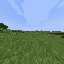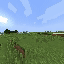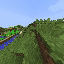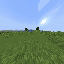After spawning in a plains biome, explore and find a cave. When inside a cave, throw a snowball to end episode.

### Observation Space¶

```Dict({
"equipped_items": {
"mainhand": {
"damage": "Box(low=-1, high=1562, shape=())",
"maxDamage": "Box(low=-1, high=1562, shape=())",
"type": "Enum(air,bucket,carrot,cobblestone,fence,fence_gate,none,other,snowball,stone_pickaxe,stone_shovel,water_bucket,wheat,wheat_seeds)"
}
},
"inventory": {
"bucket": "Box(low=0, high=2304, shape=())",
"carrot": "Box(low=0, high=2304, shape=())",
"cobblestone": "Box(low=0, high=2304, shape=())",
"fence": "Box(low=0, high=2304, shape=())",
"fence_gate": "Box(low=0, high=2304, shape=())",
"snowball": "Box(low=0, high=2304, shape=())",
"stone_pickaxe": "Box(low=0, high=2304, shape=())",
"stone_shovel": "Box(low=0, high=2304, shape=())",
"water_bucket": "Box(low=0, high=2304, shape=())",
"wheat": "Box(low=0, high=2304, shape=())",
"wheat_seeds": "Box(low=0, high=2304, shape=())"
},
"pov": "Box(low=0, high=255, shape=(64, 64, 3))"
})```

### Action Space¶

```Dict({
"attack": "Discrete(2)",
"back": "Discrete(2)",
"camera": "Box(low=-180.0, high=180.0, shape=(2,))",
"equip": "Enum(air,bucket,carrot,cobblestone,fence,fence_gate,none,other,snowball,stone_pickaxe,stone_shovel,water_bucket,wheat,wheat_seeds)",
"forward": "Discrete(2)",
"jump": "Discrete(2)",
"left": "Discrete(2)",
"right": "Discrete(2)",
"sneak": "Discrete(2)",
"sprint": "Discrete(2)",
"use": "Discrete(2)"
})```

### Starting Inventory¶

```Dict({
"snowball": 1
})```

### Usage¶

```import gym
import minerl

# Run a random agent through the environment
env = gym.make("MineRLBasaltFindCave-v0")  # A MineRLBasaltFindCave-v0 env

obs = env.reset()
done = False

while not done:
# Take a no-op through the environment.
obs, rew, done, _ = env.step(env.action_space.noop())
# Do something

######################################

# Sample some data from the dataset!
data = minerl.data.make("MineRLBasaltFindCave-v0")

# Iterate through a single epoch using sequences of at most 32 steps
for obs, rew, done, act in data.batch_iter(num_epochs=1, batch_size=32):
# Do something
```

## MineRLBasaltMakeWaterfall-v0¶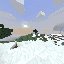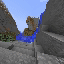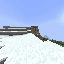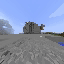After spawning in an extreme hills biome, use your waterbucket to make an beautiful waterfall. Then take an aesthetic “picture” of it by moving to a good location, positioning player’s camera to have a nice view of the waterfall, and throwing a snowball. Throwing the snowball ends the episode.

### Observation Space¶

```Dict({
"equipped_items": {
"mainhand": {
"damage": "Box(low=-1, high=1562, shape=())",
"maxDamage": "Box(low=-1, high=1562, shape=())",
"type": "Enum(air,bucket,carrot,cobblestone,fence,fence_gate,none,other,snowball,stone_pickaxe,stone_shovel,water_bucket,wheat,wheat_seeds)"
}
},
"inventory": {
"bucket": "Box(low=0, high=2304, shape=())",
"carrot": "Box(low=0, high=2304, shape=())",
"cobblestone": "Box(low=0, high=2304, shape=())",
"fence": "Box(low=0, high=2304, shape=())",
"fence_gate": "Box(low=0, high=2304, shape=())",
"snowball": "Box(low=0, high=2304, shape=())",
"stone_pickaxe": "Box(low=0, high=2304, shape=())",
"stone_shovel": "Box(low=0, high=2304, shape=())",
"water_bucket": "Box(low=0, high=2304, shape=())",
"wheat": "Box(low=0, high=2304, shape=())",
"wheat_seeds": "Box(low=0, high=2304, shape=())"
},
"pov": "Box(low=0, high=255, shape=(64, 64, 3))"
})```

### Action Space¶

```Dict({
"attack": "Discrete(2)",
"back": "Discrete(2)",
"camera": "Box(low=-180.0, high=180.0, shape=(2,))",
"equip": "Enum(air,bucket,carrot,cobblestone,fence,fence_gate,none,other,snowball,stone_pickaxe,stone_shovel,water_bucket,wheat,wheat_seeds)",
"forward": "Discrete(2)",
"jump": "Discrete(2)",
"left": "Discrete(2)",
"right": "Discrete(2)",
"sneak": "Discrete(2)",
"sprint": "Discrete(2)",
"use": "Discrete(2)"
})```

### Starting Inventory¶

```Dict({
"cobblestone": 20,
"snowball": 1,
"stone_pickaxe": 1,
"stone_shovel": 1,
"water_bucket": 1
})```

### Usage¶

```import gym
import minerl

# Run a random agent through the environment
env = gym.make("MineRLBasaltMakeWaterfall-v0")  # A MineRLBasaltMakeWaterfall-v0 env

obs = env.reset()
done = False

while not done:
# Take a no-op through the environment.
obs, rew, done, _ = env.step(env.action_space.noop())
# Do something

######################################

# Sample some data from the dataset!
data = minerl.data.make("MineRLBasaltMakeWaterfall-v0")

# Iterate through a single epoch using sequences of at most 32 steps
for obs, rew, done, act in data.batch_iter(num_epochs=1, batch_size=32):
# Do something
```

## MineRLBasaltCreateVillageAnimalPen-v0¶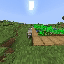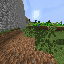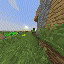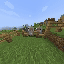After spawning in a plains village, surround two or more animals of the same type in a fenced area (a pen), constructed near the house. You can’t have more than one type of animal in your enclosed area. Allowed animals are chickens, sheep, cows, and pigs.

Do not harm villagers or existing village structures in the process.

Throw a snowball to end the episode.

### Observation Space¶

```Dict({
"equipped_items": {
"mainhand": {
"damage": "Box(low=-1, high=1562, shape=())",
"maxDamage": "Box(low=-1, high=1562, shape=())",
"type": "Enum(air,bucket,carrot,cobblestone,fence,fence_gate,none,other,snowball,stone_pickaxe,stone_shovel,water_bucket,wheat,wheat_seeds)"
}
},
"inventory": {
"bucket": "Box(low=0, high=2304, shape=())",
"carrot": "Box(low=0, high=2304, shape=())",
"cobblestone": "Box(low=0, high=2304, shape=())",
"fence": "Box(low=0, high=2304, shape=())",
"fence_gate": "Box(low=0, high=2304, shape=())",
"snowball": "Box(low=0, high=2304, shape=())",
"stone_pickaxe": "Box(low=0, high=2304, shape=())",
"stone_shovel": "Box(low=0, high=2304, shape=())",
"water_bucket": "Box(low=0, high=2304, shape=())",
"wheat": "Box(low=0, high=2304, shape=())",
"wheat_seeds": "Box(low=0, high=2304, shape=())"
},
"pov": "Box(low=0, high=255, shape=(64, 64, 3))"
})```

### Action Space¶

```Dict({
"attack": "Discrete(2)",
"back": "Discrete(2)",
"camera": "Box(low=-180.0, high=180.0, shape=(2,))",
"equip": "Enum(air,bucket,carrot,cobblestone,fence,fence_gate,none,other,snowball,stone_pickaxe,stone_shovel,water_bucket,wheat,wheat_seeds)",
"forward": "Discrete(2)",
"jump": "Discrete(2)",
"left": "Discrete(2)",
"right": "Discrete(2)",
"sneak": "Discrete(2)",
"sprint": "Discrete(2)",
"use": "Discrete(2)"
})```

### Starting Inventory¶

```Dict({
"carrot": 1,
"fence": 64,
"fence_gate": 64,
"snowball": 1,
"wheat": 1,
"wheat_seeds": 1
})```

### Usage¶

```import gym
import minerl

# Run a random agent through the environment
env = gym.make("MineRLBasaltCreateVillageAnimalPen-v0")  # A MineRLBasaltCreateVillageAnimalPen-v0 env

obs = env.reset()
done = False

while not done:
# Take a no-op through the environment.
obs, rew, done, _ = env.step(env.action_space.noop())
# Do something

######################################

# Sample some data from the dataset!
data = minerl.data.make("MineRLBasaltCreateVillageAnimalPen-v0")

# Iterate through a single epoch using sequences of at most 32 steps
for obs, rew, done, act in data.batch_iter(num_epochs=1, batch_size=32):
# Do something
```

## MineRLBasaltBuildVillageHouse-v0¶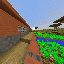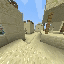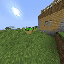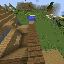Build a house in the style of the village without damaging the village. Give a tour of the house and then throw a snowball to end the episode.

Note

In the observation and action spaces, the following (internal Minecraft) item IDs can be interpreted as follows:

• `log#0` is oak logs.

• `log#1` is spruce logs.

• `log2#0` is acacia logs.

• `planks#0` is oak planks.

• `planks#1` is spruce planks.

• `planks#4` is acacia planks.

• `sandstone#0` is cracked sandstone.

• `sandstone#2` is smooth sandstone.

Tip

You can find detailed information on which materials are used in each biome-specific village (plains, savannah, taiga, desert) here: https://minecraft.fandom.com/wiki/Village/Structure_(old)/Blueprints#Village_generation

### Observation Space¶

```Dict({
"equipped_items": {
"mainhand": {
"damage": "Box(low=-1, high=1562, shape=())",
"maxDamage": "Box(low=-1, high=1562, shape=())",
}
},
"inventory": {
"acacia_door": "Box(low=0, high=2304, shape=())",
"acacia_fence": "Box(low=0, high=2304, shape=())",
"cactus": "Box(low=0, high=2304, shape=())",
"cobblestone": "Box(low=0, high=2304, shape=())",
"dirt": "Box(low=0, high=2304, shape=())",
"fence": "Box(low=0, high=2304, shape=())",
"flower_pot": "Box(low=0, high=2304, shape=())",
"glass": "Box(low=0, high=2304, shape=())",
"log#0": "Box(low=0, high=2304, shape=())",
"log#1": "Box(low=0, high=2304, shape=())",
"log2#0": "Box(low=0, high=2304, shape=())",
"planks#0": "Box(low=0, high=2304, shape=())",
"planks#1": "Box(low=0, high=2304, shape=())",
"planks#4": "Box(low=0, high=2304, shape=())",
"red_flower": "Box(low=0, high=2304, shape=())",
"sand": "Box(low=0, high=2304, shape=())",
"sandstone#0": "Box(low=0, high=2304, shape=())",
"sandstone#2": "Box(low=0, high=2304, shape=())",
"sandstone_stairs": "Box(low=0, high=2304, shape=())",
"snowball": "Box(low=0, high=2304, shape=())",
"spruce_door": "Box(low=0, high=2304, shape=())",
"spruce_fence": "Box(low=0, high=2304, shape=())",
"stone_axe": "Box(low=0, high=2304, shape=())",
"stone_pickaxe": "Box(low=0, high=2304, shape=())",
"stone_stairs": "Box(low=0, high=2304, shape=())",
"torch": "Box(low=0, high=2304, shape=())",
"wooden_door": "Box(low=0, high=2304, shape=())",
"wooden_pressure_plate": "Box(low=0, high=2304, shape=())"
},
"pov": "Box(low=0, high=255, shape=(64, 64, 3))"
})```

### Action Space¶

```Dict({
"attack": "Discrete(2)",
"back": "Discrete(2)",
"camera": "Box(low=-180.0, high=180.0, shape=(2,))",
"forward": "Discrete(2)",
"jump": "Discrete(2)",
"left": "Discrete(2)",
"right": "Discrete(2)",
"sneak": "Discrete(2)",
"sprint": "Discrete(2)",
"use": "Discrete(2)"
})```

### Starting Inventory¶

```Dict({
"acacia_door": 64,
"acacia_fence": 64,
"cactus": 3,
"cobblestone": 64,
"dirt": 64,
"fence": 64,
"flower_pot": 3,
"glass": 64,
"log#0": 64,
"log#1": 64,
"log2#0": 64,
"planks#0": 64,
"planks#1": 64,
"planks#4": 64,
"red_flower": 3,
"sand": 64,
"sandstone#0": 64,
"sandstone#2": 64,
"sandstone_stairs": 64,
"snowball": 1,
"spruce_door": 64,
"spruce_fence": 64,
"stone_axe": 1,
"stone_pickaxe": 1,
"stone_stairs": 64,
"torch": 64,
"wooden_door": 64,
"wooden_pressure_plate": 64
})```

### Usage¶

```import gym
import minerl

# Run a random agent through the environment
env = gym.make("MineRLBasaltBuildVillageHouse-v0")  # A MineRLBasaltBuildVillageHouse-v0 env

obs = env.reset()
done = False

while not done:
# Take a no-op through the environment.
obs, rew, done, _ = env.step(env.action_space.noop())
# Do something

######################################

# Sample some data from the dataset!
data = minerl.data.make("MineRLBasaltBuildVillageHouse-v0")

# Iterate through a single epoch using sequences of at most 32 steps
for obs, rew, done, act in data.batch_iter(num_epochs=1, batch_size=32):
# Do something
```